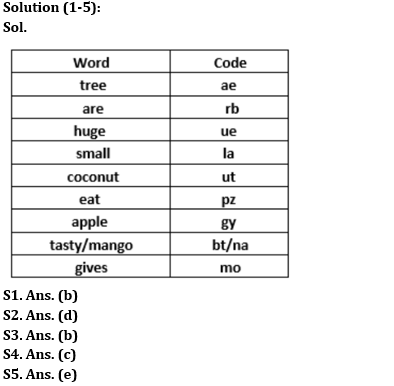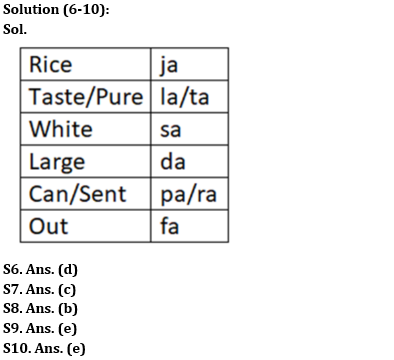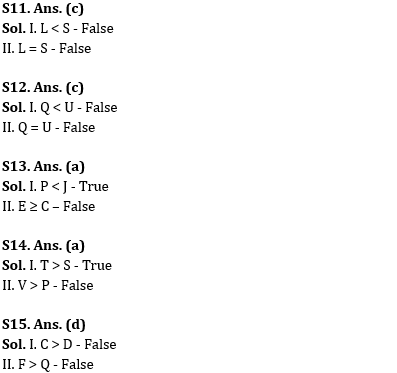Latest Banking jobs   »

# Reasoning Ability Quiz For IBPS RRB PO Prelims 2022- 15th June

Directions (1-5): Study the following information carefully and answer the questions accordingly.
In a certain code language
‘Apple eat small tree gives’ is written as ‘pz mo la ae gy’,
‘Gives are tasty eat mango’ is written as ‘na rb bt pz mo’,
‘Tasty mango tree are huge’ is written as ‘rb ae ue na bt’,
‘Eat huge coconut are small’ is written as ‘la rb ut pz ue’.

Q1. Which words are coded as ‘ue’ and ‘la’?
(a) Are and small
(b) Huge and small
(c) Are and eat
(d) Huge and eat
(e) None of these

Q2. What is the code for ‘gives’?
(a) Either ‘ae’ or ‘mo’
(b) ae
(c) pz
(d) mo
(e) Cannot be determined

Q3. If the code for ‘mango’ gets interchanged with the code for ‘tree’ and then ‘coconut tree fruits’ is coded as ‘ts na ut’, find what will be the possible code for ‘tasty fruits are good’?
(c) ts rb mo pz
(e) rb ue na ae

Q4. What is the code for ‘gy ae mo we’?
(a) Apple coconut gives gold
(b) Tree gives bitter sweet
(c) Tree gives sweet apple
(d) Apple tree gives tasty
(e) Small tree gives apple

Q5. What will be the code for ‘tasty coconut’?
(a) ut na
(b) ut bt
(c) ue ut
(d) ae na
(e) Can’t be determined

Directions (6-10): Study the following information carefully and answer the questions accordingly.
In a certain code language,
‘Rice taste pure white’ is coded as ‘la ta ja sa’
‘Large can sent rice’ is coded as ‘ja pa ra da’
‘Out taste pure large’ is coded as ‘da ta fa la’

Q6. What is the code for ‘out’?
(a) la
(b) ta
(c) da
(d) fa
(e) None of these

Q7. Which of the following is coded as ‘sa’?
(a) Rice
(b) Pure
(c) White
(d) Taste
(e) None of these

Q8. What will be the possible code for ‘pure large’?
(a) la sa
(b) ta da
(c) pa ta
(d) ra fa
(e) ja da

Q9. Which of the following is coded as ‘La’?
(a) Rice
(b) Pure
(c) White
(d) Taste
(e) Can’t be determined

Q10. What is the code for ‘Can’?
(a) la
(b) pa
(c) da
(d) ra
(e) Either ‘pa’ or ‘ra’

Directions (11-15): In the following questions assuming the given statement to be true, find which of the conclusion(s) among given conclusions is/are definitely true and then give your answers accordingly.

Q11. Statements: D = E ≤ S, A = L ≤ C ≤ D, S ≥ G > H
Conclusions:
I. L < S
II. L = S
(a) Only I is true
(b) Only II is true
(c) Either I or II is true
(d) Neither I nor II is true
(e) Both I and II are true

Q12. Statements: R ≤ O = U ≥ Y, P = Q = R ≤ R, Y = W > X
Conclusions:
I. Q < U
II. Q = U
(a) Only I is true
(b) Only II is true
(c) Either I or II is true
(d) Neither I nor II is true
(e) Both I and II are true

Q13. Statements: B ≥ P < H ≤ C, D > E = B, C < J > K ≥ L
Conclusions:
I. P < J
II. E ≥ C
(a) Only I is true
(b) Only II is true
(c) Either I or II is true
(d) Neither I nor II is true
(e) Both I and II are true

Q14. Statements: V < X, T = O, X ≤ P, O ≥ V > S = H
Conclusions:
I. T > S
II. V > P
(a) Only I is true
(b) Only II is true
(c) Either I or II is true
(d) Neither I nor II is true
(e) Both I and II are true

Q15. Statements: Q = W > D, E ≤ F, C < W, C ≤ F
Conclusions:
I. C > D
II. F > Q
(a) Only I is true
(b) Only II is true
(c) Either I or II is true
(d) Neither I nor II is true
(e) Both I and II are true

Solutions#### Congratulations!